In this article, we will see how to create and use the .Net class library (DLL) in C# using visual studio. A class library is a collection of class definitions contained in a *.DLL or *.Exe format. We can easily use the class library in any visual studio project.
• 5.7k
• 0
• 0

In this article, we will see how to create and use the .Net class library (DLL) in C# using visual studio. A class library is a collection of class definitions contained in a *.DLL or *.Exe format. We can easily use the class library in any visual studio project.

• Creating a Class Library (DLL) in C#
• Using the Class Library in other Visual Studio Project

## 1. Creating a Class Library (DLL) in C#

First, we will create a class library project using visual studio and add the math function methods to it.

## Step 1:

Open Visual Studio 2019 and click "Create a new project" and choose Class Library (.Net Framework).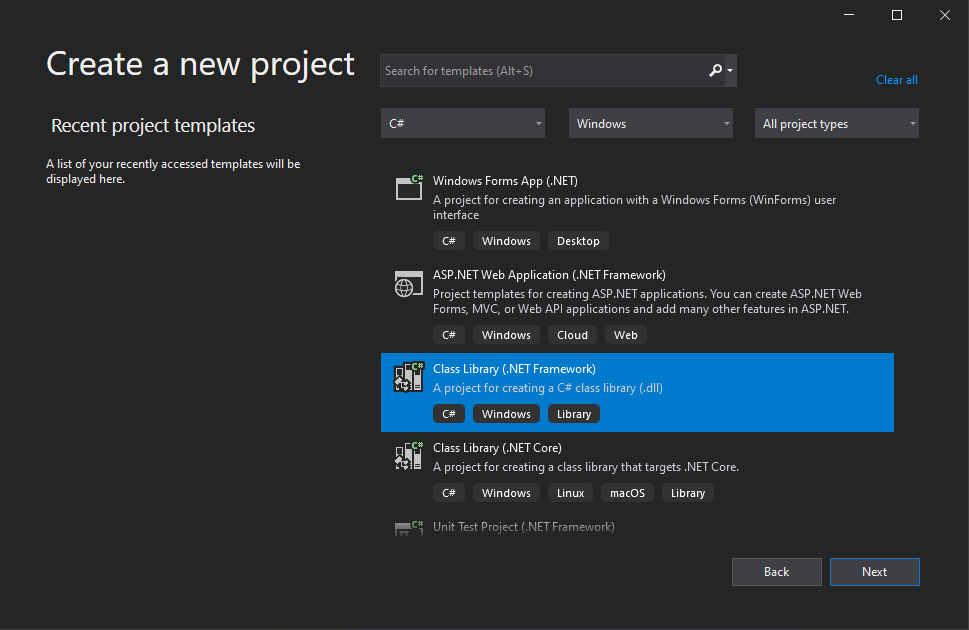Provide the project name and location and click "Create" as shown below,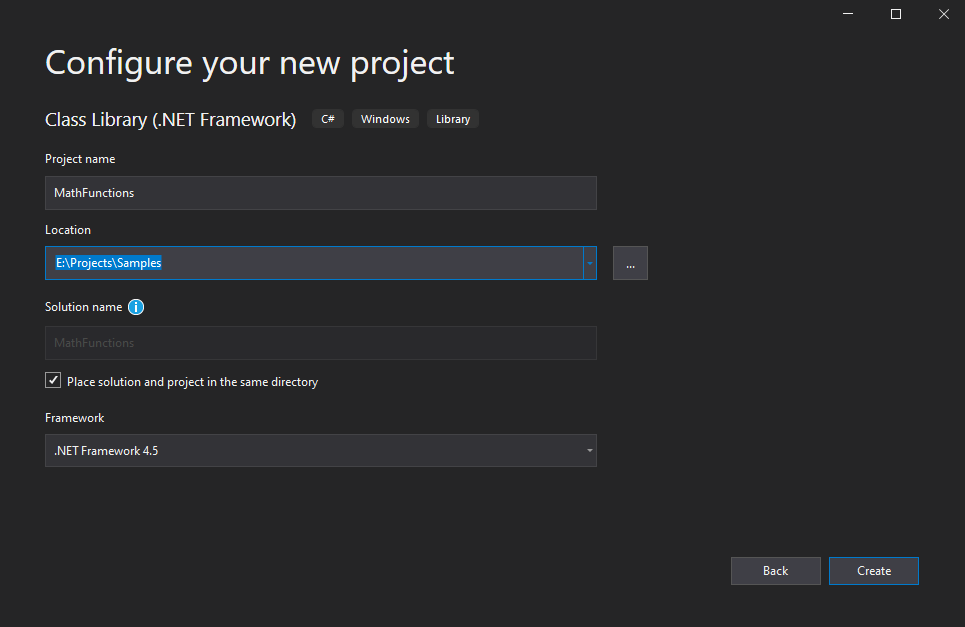Once the class library project is created, you will see the below file structure. Add class file "Functions.cs" or rename "Class1.cs" to "Functions.cs"## Step 2: Adding math functions

Add below math functions (Add, Subtract, Multiply and Divide) to the class file as shown below,

``````using System;

namespace MathFunctions
{
public class Functions
{
/// <summary>
/// </summary>
/// <param name="a"></param>
/// <param name="b"></param>
/// <returns></returns>
public int Add (int a, int b)
{
return a + b;
}

/// <summary>
/// Substract Function
/// </summary>
/// <param name="a"></param>
/// <param name="b"></param>
/// <returns></returns>
public int Substract(int a, int b)
{
return a - b;
}

/// <summary>
/// Multiply Function
/// </summary>
/// <param name="a"></param>
/// <param name="b"></param>
/// <returns></returns>
public int Multiply(int a, int b)
{
return a * b;
}

/// <summary>
/// Divide Function
/// </summary>
/// <param name="a"></param>
/// <param name="b"></param>
/// <returns></returns>
public int Divide(int a, int b)
{
return a / b;
}
}
}
``````

## Step 3:

Next, build the class library project and see the bin folder of the application for the DLL as shown below,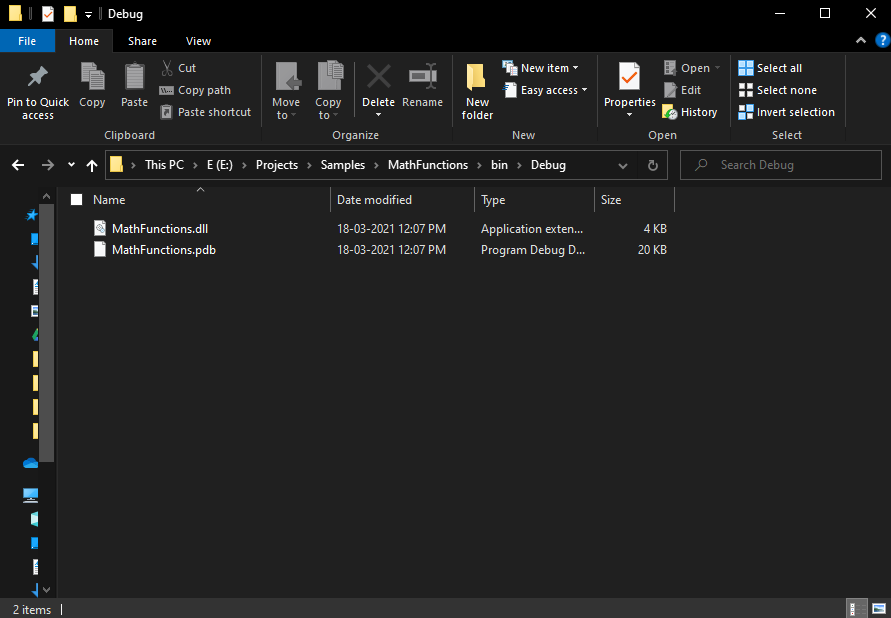Now, we are ready to consume this class library in other visual studio projects.

## Step 1:

Open Visual Studio 2019 and click "Create a new project" and choose Console App (.Net Framework).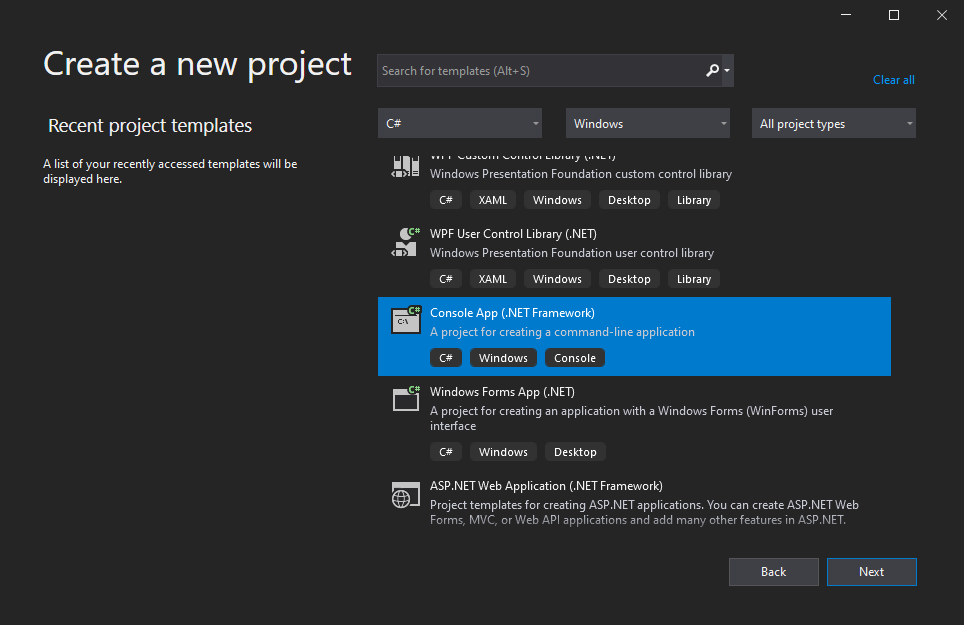Provide the project name and location and click "Create" as shown below,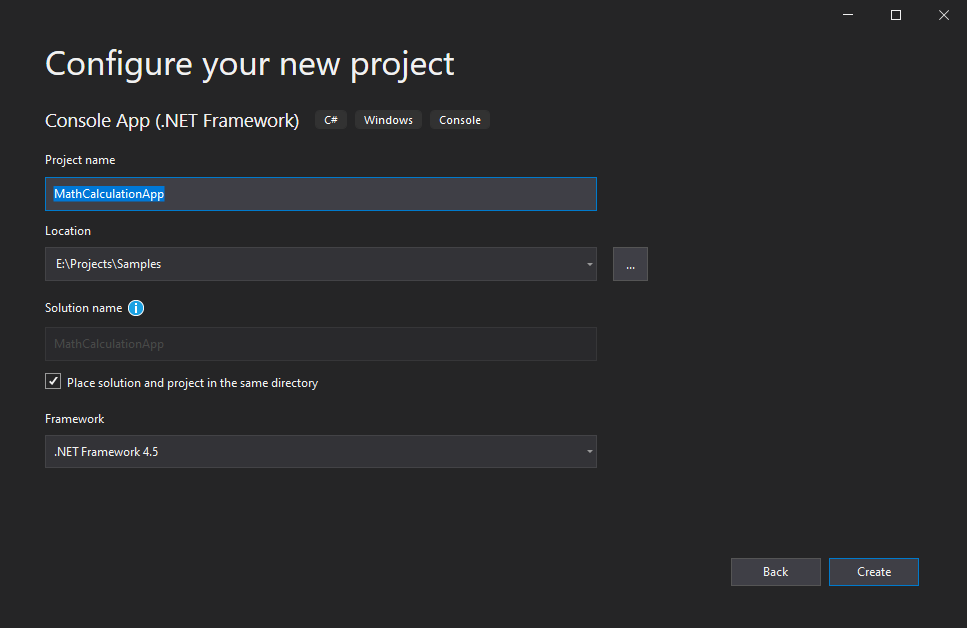## Step 2: Adding "MathFunctions.DLL" as a reference

To add reference "MathFunctions.DLL", right-click the "References"  tab and choose the "Add Reference" option as shown below,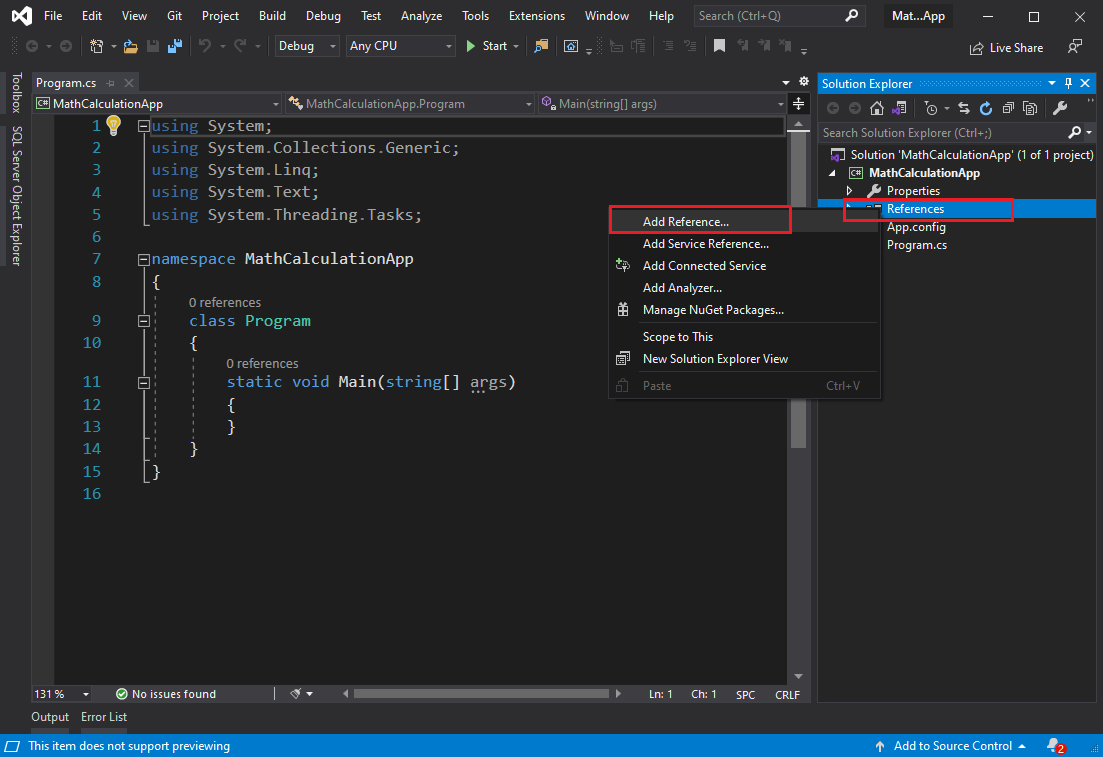## Step 3:

The "Reference Manager" will appear, then you have to choose the "Browse" tab on the left side of the dialog and then click "Browse" in the dialog as shown below,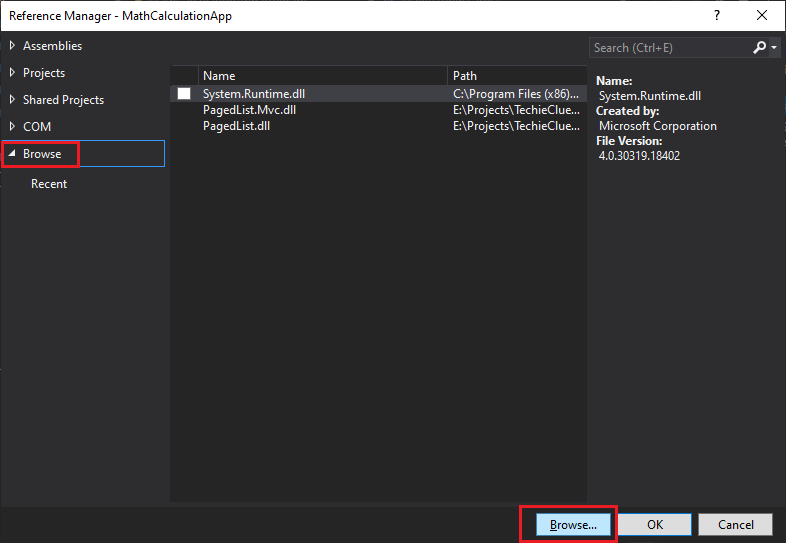The below dialog will appear to select the DLL, (you have to go to your class library bin folder to choose the DLL),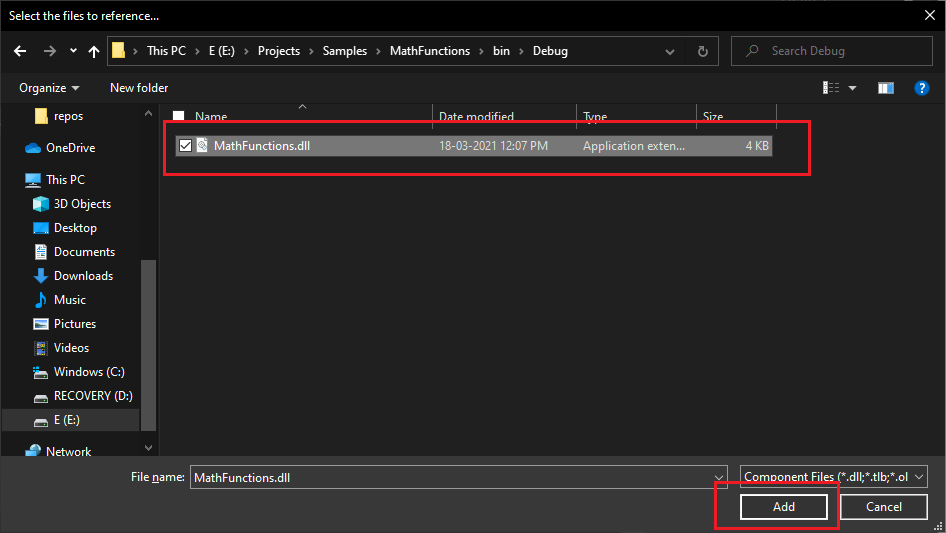In the above dialog, Select the "MathFunctions.dll" and click the "Add" button to add the DLL as a reference in our project.

You can see the "MathFunctions" reference in the "References" tab in your project now.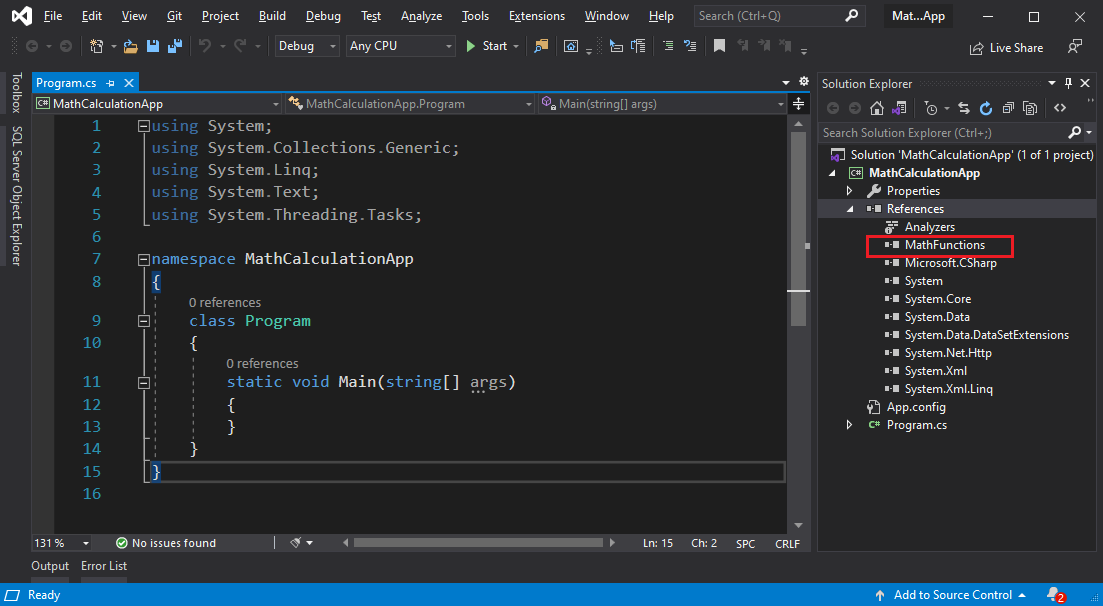## Step 4:

Next, we have to add the "MathFunctions" namespace (`using MathFunctions;`) as shown below,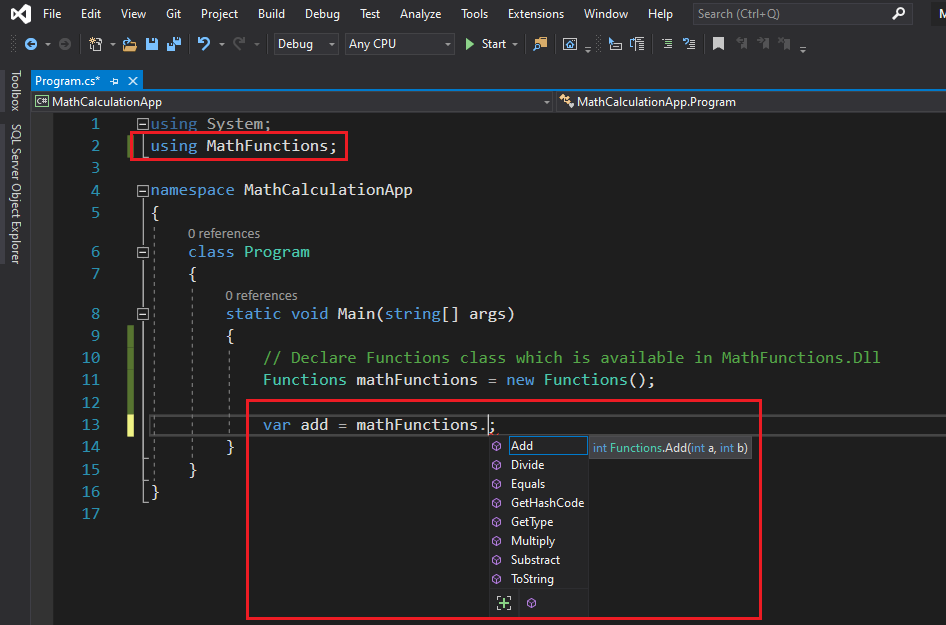You can now access all the math function methods inside the "Functions" class after the declaration (see above).

## Step 5:

Add the below code to access all the math functions from the class library (DLL).

``````using System;
using MathFunctions;

namespace MathCalculationApp
{
class Program
{
static void Main(string[] args)
{
// Declare Functions class which is available in MathFunctions.Dll
Functions mathFunctions = new Functions();

// Access Add method from the class library

// Access Substract from the class library
var substract = mathFunctions.Substract(20, 10);
Console.WriteLine("Substract : " + subtract);   // Print value

// Access Multiply from the class library
var multiply = mathFunctions.Multiply(20, 10);
Console.WriteLine("Multiply : " + multiply);   // Print value

// Access Divide from the class library
var divide = mathFunctions.Divide(20, 10);
Console.WriteLine("Divide : " + divide);    // Print value

}
}
}
``````

## Output:

Build and run your application and see the output as shown below,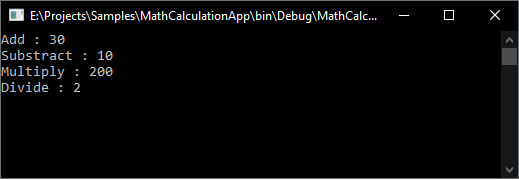Sabari M
Software Professional, India

IT professional with 15+ years of experience in Microsoft Technologies with a strong base in Microsoft .NET (C#.Net, ASP.Net MVC, ASP.NET WEB API, Webservices,...Read More

https://www.techieclues.com/profile/alagu-mano-sabari-m